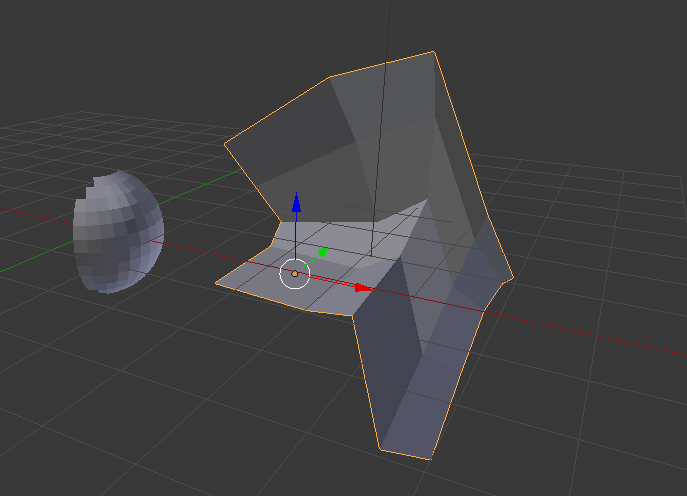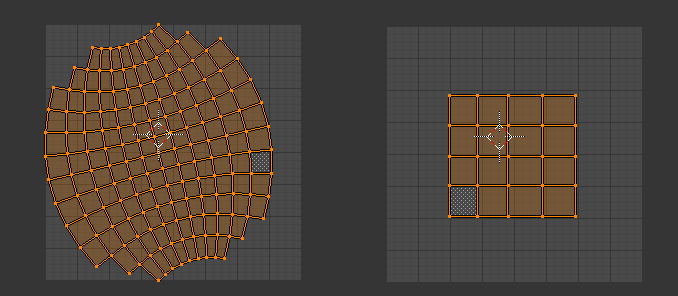For certain reasons, which are hard to explain I needed a way to deform a given mesh to match the surface of another mesh, while its internal topology is maintained. I found the best way for me to do this, is define the mapping via the overlap in UV. So here is the type of problem I facedHow to bring the right mesh into the form of the left mesh? Maybe, there is already a function for that but I found it easier to simply unwrap both meshes and define where the uv-area should overlapSo the uv-map of the right mesh is somewhere within the uv-map fo the left mesh. Then hit “Map via UV” and the result isHere is the add-on. I hope it is useful for some of you.

``````
bl_info = {
"name": "Map via UV",
"description": "Maps a mesh on the form of another mesh via UV-overlap",
"author": "Martin Pyka",
"version": (1, 0),
"blender": (2, 70, 0),
"location": "View3D &gt; Add &gt; Mesh",
"warning": "", # used for warning icon and text in addons panel
"wiki_url": "http://www.martinpyka.de",
"category": "Mesh"}

import bpy
import mathutils

def main(context):
for ob in context.scene.objects:
print(ob)

class MapUVOperator(bpy.types.Operator):
"""Tooltip"""
bl_idname = "object.map_via_uv"
bl_label = "Map via UV overlap"
bl_description = "Maps a mesh on the form of another mesh via UV-overlap"

@classmethod
def poll(cls, context):
active_obj = context.active_object
if active_obj is not None:
return active_obj.type == "MESH"
else:
return False

def execute(self, context):
target_obj = context.active_object
if (bpy.context.selected_objects.index(target_obj) == 0):
origin_obj = bpy.context.selected_objects
else:
origin_obj = bpy.context.selected_objects

map_via_uv(origin_obj, target_obj)

return {'FINISHED'}

def invoke(self, context, event):

return self.execute(context)

def register():
bpy.utils.register_class(MapUVOperator)

def unregister():
bpy.utils.unregister_class(MapUVOperator)

if __name__ == "__main__":
register()

# test call
# bpy.ops.object.simple_operator()

# TODO(SK): Quads into triangles (indices)
def map3dPointToUV(object, object_uv, point, normal=None):
"""Converts a given 3d-point into uv-coordinates,
object for the 3d point and object_uv must have the same topology
if normal is not None, the normal is used to detect the point on object, otherwise
the closest_point_on_mesh operation is used
"""

# if normal is None, we don't worry about orthogonal projections
if normal is None:
# get point, normal and face of closest point to a given point
p, n, f = object.closest_point_on_mesh(point)
else:
p, n, f = object.ray_cast(point + normal * config.ray_fac, point - normal * config.ray_fac)
# if no collision could be detected, return None
if f == -1:
return None

# get the uv-coordinate of the first triangle of the polygon
A = object.data.vertices[object.data.polygons[f].vertices].co
B = object.data.vertices[object.data.polygons[f].vertices].co
C = object.data.vertices[object.data.polygons[f].vertices].co

# and the uv-coordinates of the first triangle
uvs = [object_uv.data.uv_layers.active.data[li] for li in object_uv.data.polygons[f].loop_indices]
U = uvs.uv.to_3d()
V = uvs.uv.to_3d()
W = uvs.uv.to_3d()

# convert 3d-coordinates of point p to uv-coordinates
p_uv = mathutils.geometry.barycentric_transform(p, A, B, C, U, V, W)

# if the point is not within the first triangle, we have to repeat the calculation
# for the second triangle
if (mathutils.geometry.intersect_point_tri_2d(p_uv.to_2d(), uvs.uv, uvs.uv, uvs.uv) == 0) & (len(uvs)==4):
A = object.data.vertices[object.data.polygons[f].vertices].co
B = object.data.vertices[object.data.polygons[f].vertices].co
C = object.data.vertices[object.data.polygons[f].vertices].co

U = uvs.uv.to_3d()
V = uvs.uv.to_3d()
W = uvs.uv.to_3d()

p_uv = mathutils.geometry.barycentric_transform(p, A, B, C, U, V, W)

return p_uv.to_2d()

# TODO(SK): Quads into triangles (indices)
def mapUVPointTo3d(object_uv, uv_list, cleanup=True):
""" Converts a list of uv-points into 3d. This function is mostly
used by interpolateUVTrackIn3D. Note, that therefore, not all points
can and have to be converted to 3d points. The return list can therefore
have less points than the uv-list. This cleanup can be deactivated
by setting cleanup = False. Then, the return-list may contain
some [] elements.
"""

uv_polygons = []

points_3d = [[] for j in range(len(uv_list))]
to_find = [i for i in range(len(uv_list))]

for p in uv_polygons:
uvs = [object_uv.data.uv_layers.active.data[li] for li in p.loop_indices]
for i in to_find:
result = mathutils.geometry.intersect_point_tri_2d(
uv_list[i],
uvs.uv,
uvs.uv,
uvs.uv
)
if (result != 0):
U = uvs.uv.to_3d()
V = uvs.uv.to_3d()
W = uvs.uv.to_3d()
A = object_uv.data.vertices[p.vertices].co
B = object_uv.data.vertices[p.vertices].co
C = object_uv.data.vertices[p.vertices].co
points_3d[i] = mathutils.geometry.barycentric_transform(uv_list[i].to_3d(), U, V, W, A, B, C)
to_find.remove(i)
else:
result = mathutils.geometry.intersect_point_tri_2d(
uv_list[i],
uvs.uv,
uvs.uv,
uvs.uv
)
if (result != 0):
U = uvs.uv.to_3d()
V = uvs.uv.to_3d()
W = uvs.uv.to_3d()
A = object_uv.data.vertices[p.vertices].co
B = object_uv.data.vertices[p.vertices].co
C = object_uv.data.vertices[p.vertices].co
points_3d[i] = mathutils.geometry.barycentric_transform(uv_list[i].to_3d(), U, V, W, A, B, C)
to_find.remove(i)
if len(to_find) == 0:
return points_3d

for p in object_uv.data.polygons:
uvs = [object_uv.data.uv_layers.active.data[li] for li in p.loop_indices]
to_delete = []
for i in to_find:
result = mathutils.geometry.intersect_point_tri_2d(
uv_list[i],
uvs.uv,
uvs.uv,
uvs.uv
)
if (result != 0):
U = uvs.uv.to_3d()
V = uvs.uv.to_3d()
W = uvs.uv.to_3d()
A = object_uv.data.vertices[p.vertices].co
B = object_uv.data.vertices[p.vertices].co
C = object_uv.data.vertices[p.vertices].co
points_3d[i] = mathutils.geometry.barycentric_transform(uv_list[i].to_3d(), U, V, W, A, B, C)
to_delete.append(i)
uv_polygons.append(p)
else:
result = mathutils.geometry.intersect_point_tri_2d(
uv_list[i],
uvs.uv,
uvs.uv,
uvs.uv
)
if (result != 0):
U = uvs.uv.to_3d()
V = uvs.uv.to_3d()
W = uvs.uv.to_3d()
A = object_uv.data.vertices[p.vertices].co
B = object_uv.data.vertices[p.vertices].co
C = object_uv.data.vertices[p.vertices].co
points_3d[i] = mathutils.geometry.barycentric_transform(uv_list[i].to_3d(), U, V, W, A, B, C)
to_delete.append(i)
uv_polygons.append(p)
if len(to_find) == 0:
return points_3d

for d in to_delete:
to_find.remove(d)

if cleanup:
points_3d = [p for p in points_3d if p != []]

return points_3d

def map_via_uv(origin, target):
""" Maps a mesh on the surface of another mesh while preserving
its topology. The mapping uses the uv-space
origin          : mesh to map
target          : mesh-form that is targeted
"""

if not origin.type == "MESH":
raise Exception("Origin object is not a mesh")

if not target.type == "MESH":
raise Exception("Target object is not a mesh")

o_2d = []

for v in origin.data.vertices:
o_2d.append(
map3dPointToUV(origin, origin, v.co)
)

t_3d = mapUVPointTo3d(target, o_2d)

if len(t_3d) != len(o_2d):
raise Exception("One of the points is not in the UV-grid of the " +
"target object")

for i in range(len(t_3d)):
origin.data.vertices[i].co = t_3d[i]

``````

do you have only quads in your mesh? I guess, this routine assumes that everything consists of quads.

It is kind of tricky to get it working. At least for me…

There are two mesh objects, which should be all quads.

Let’s call one the target mesh and the other the conforming mesh.

Have a 3D editor and a UV editor (aka image editor) panel / window open to do this. It is not clear the thread but the steps are:

1. select the target mesh and enter edit

2. unwrap the target mesh (like with smart UV or sphere unwrap)

3. select, move or resize UV points until all are enclosed within the bounds of the background grid in the UV editor.

4. select a region of UVs desired (target UVs)

5. note where the UVs are and their size (IMPORTANT!)

6. leave edit mode

7. repeat steps 1 - 4 now for the conforming mesh

8. with the UVs desired selected (conforming UVs), move and scale them to make sure they are within the desired region of UVs of the target mesh such that there is no overlap.

The conforming UVs should cover the same area or be completely within the bounds of the target UVs otherwise an error ‘One of the points is not in the UV-grid of the target object’ occurs.

Interestingly, if the conforming mesh is a tri mesh, and the target mesh a quad, the same error can occur if conforming UVs are enclosing target UVs

Otherwise, if the target mesh is a triangulated mesh, an error ‘list index out of range’ occurs. The target mesh should be a quad mesh.

1. repeat step 5. All meshes should not be in edit mode

2. select both conforming mesh and target mesh in that order

3. press spacebar, select ‘search’, type in ‘map via uv overlap’ and click on the matching option

4. Repeat step 11 to vary results

Hint:
MultiEdit addon can be a big help
Ctrl-L is great too in the UV editor when selecting all UVs of a mesh from just one selected UV point!